Find the area of the figure:

# Find the area of the figure: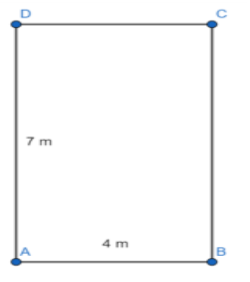1. A
20
2. B
42
3. C
14
4. D
28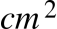Fill Out the Form for Expert Academic Guidance!l

+91

Live ClassesBooksTest SeriesSelf Learning

Verify OTP Code (required)

### Solution:

Concept- We have a rectangle, and the graphic also shows the rectangle's measurements. We must apply the formula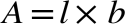, where l is the rectangle's length and b is its width, to get the area of the rectangle.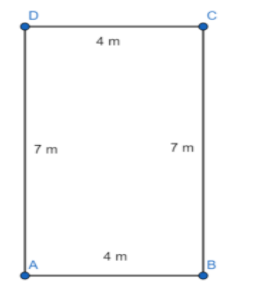As a result, we may depict the resulting rectangle as in the image above.
Therefore, it is evident that the rectangle's width is equal to 4 meters and its length is equal to 7 meters.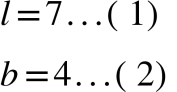We are aware that if a rectangle's length and width are both equal to l and b, then the area of the rectangle is equal to the sum of l and b.
Assume that A is the same as the rectangle's area.When we replace equations (1) and (2) in equation (3) , we obtain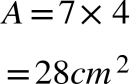Hence, the correct option is 4) 28## Related content

 Area of Square Area of Isosceles Triangle Pythagoras Theorem Triangle Formula Perimeter of Triangle Formula Area Formulae Volume of Cone Formula Matrices and Determinants_mathematics Critical Points Solved Examples Type of relations_mathematics+91

Live ClassesBooksTest SeriesSelf Learning

Verify OTP Code (required)